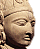xElliptic curve
Posts  1 - 1  of  1jangbsung
I am trying to prove that, in the following
equation, we cannot have natural numbers
that satisfy it.

C, D , and E are prime to each other.
Also,
P, Q, R, T, and U are prime to each other.
*; multiply
^' exponent

{ (P^2+PR+R^2)*(Q^2+QS+S^2) * (T^2 + TU + U^2) } ^3

= { (C^2+CD+D^2)*(C^2 + CE + E^2) * (D^2 - DE * E^2) } ^ 2

These forms are called CYCLOTOMES.

Is it possible to prove the above claim
using elliptic curve theory?